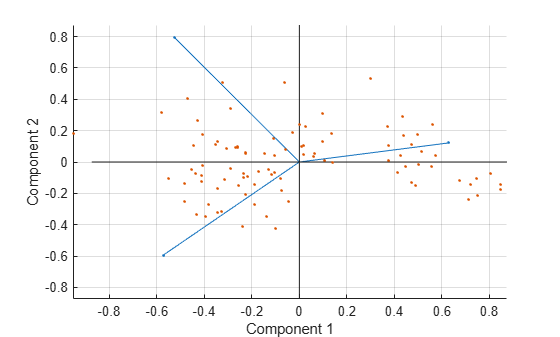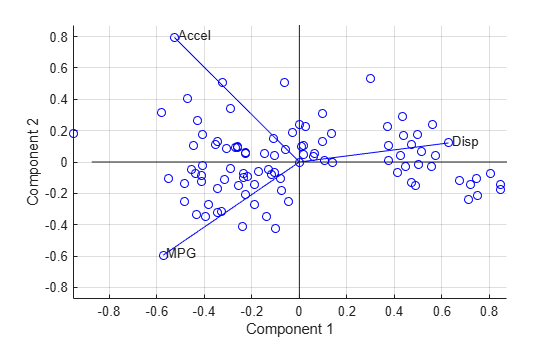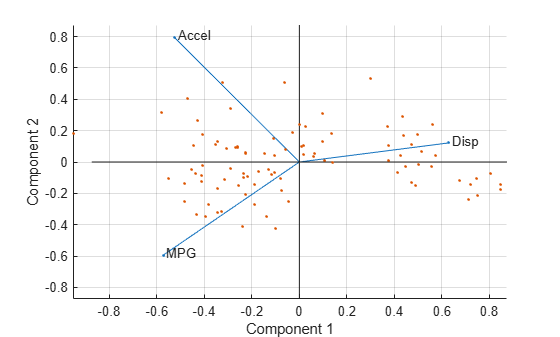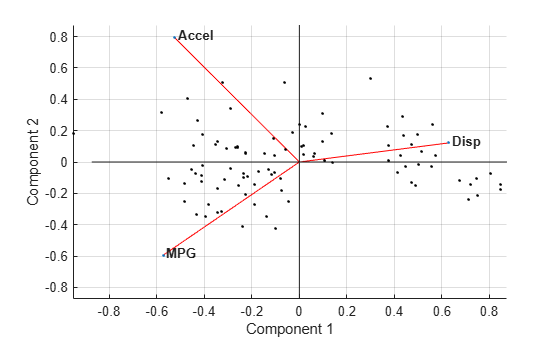# biplot

## Syntax

``biplot(coefs)``
``biplot(coefs,Name,Value)``
``biplot(ax,___)``
``h = biplot(___)``

## Description

example

````biplot(coefs)` creates a biplot of the coefficients in the matrix `coefs`. The biplot is 2-D if `coefs` has two columns or 3-D if it has three columns. The axes in the biplot represent the columns of `coefs`, and the vectors in the biplot represent the rows of `coefs` (the observed variables).```

example

````biplot(coefs,Name,Value)` specifies additional options using one or more name-value pair arguments. For example, you can specify `'Positive','true'` to restrict the biplot to the positive quadrant (in 2-D) or octant (in 3-D).```

example

````biplot(ax,___)` uses the plot axes specified by the `Axes` object `ax`. Specify `ax` as the first input argument followed by any of the input argument combinations in the previous syntaxes.```

example

````h = biplot(___)` returns a column vector of handles to the graphics objects created by `biplot`. Use `h` to query and modify properties of specific graphics objects. For more information, see Graphics Object Properties.```

## Examples

collapse all

Create a biplot of the first three principal component coefficients, the observations, and the observed variables for the `carsmall` data set.

`load carsmall`

Create a matrix consisting of the variables `Acceleration`, `Displacement`, `Horsepower`, `MPG`, and `Weight`. Delete rows in the matrix that have missing values.

```X = [Acceleration Displacement Horsepower MPG Weight]; X = rmmissing(X);```

Standardize `X` and perform a principal component analysis.

```Z = zscore(X); % Standardized data [coefs,score] = pca(Z);```

The 5-by-5 matrix `coefs` contains the principal component coefficients (one column for each principal component). The matrix `score` contains the principal component scores (the observations).

Create a biplot of the first three principal component coefficients. The axes of the biplot represent the columns of `coefs`, and the vectors in the biplot represent the rows of `coefs`.

`biplot(coefs(:,1:3))`Create a more detailed biplot by labeling each variable and plotting the observations in the space of the first three principal components.

```vbls = {'Accel','Disp','HP','MPG','Wgt'}; % Labels for the variables biplot(coefs(:,1:3),'Scores',score(:,1:3),'VarLabels',vbls);```Load the `fisheriris` data set, standardize the flower measurements in `meas`, and perform a principal component analysis.

```load fisheriris Z = zscore(meas); [coefs,scores] = pca(Z);```

Create a figure with two subplots and return the `Axes` objects as `ax1` and `ax2`. Create a biplot in each set of axes by referring to the corresponding `Axes` object. In the top subplot, display a biplot using the first two principal components. In the bottom subplot, display a biplot using the third and fourth principal components. Specify the x-axis and y-axis limits by passing the corresponding `Axes` objects to the `xlim` and `ylim` functions. Change the x-axis and y-axis labels in the bottom plot by passing `ax2` to `xlabel` and `ylabel`.

```figure('Units','normalized','Position',[0.3 0.3 0.3 0.5]) variables = {'SepalLength','SepalWidth','PetalLength','PetalWidth'}; ax1 = subplot(2,1,1); % Top subplot biplot(ax1,coefs(:,1:2),'Scores',scores(:,1:2),'VarLabels',variables); xlim(ax1,[-1 1]) ylim(ax1,[-1 1]) ax2 = subplot(2,1,2); % Bottom subplot biplot(ax2,coefs(:,3:4),'Scores',scores(:,3:4),'VarLabels',variables); xlim(ax2,[-1 1]) ylim(ax2,[-1 1]) xlabel(ax2,'Component 3') ylabel(ax2,'Component 4')```Control the appearance of a biplot by specifying supported line property names and values, and by using handles to the graphics objects created by `biplot`.

`load carsmall`

Create a matrix consisting of the variables `Acceleration`, `Displacement`, and `MPG`. Delete rows in the matrix that have missing values.

```X = [Acceleration Displacement MPG]; X = rmmissing(X);```

Standardize `X` and perform a principal component analysis.

```Z = zscore(X); % Standardized data [coefs,score] = pca(Z);```

The 3-by-3 matrix `coefs` contains the principal component coefficients (one column for each principal component). The matrix `score` contains the principal component scores (the observations).

Create a biplot of the observations in the space of the first two principal components. Use the default properties for the biplot.

`h = biplot(coefs(:,1:2),'Scores',score(:,1:2));``h` is a vector of handles to graphics objects. You can modify the properties of the line objects returned by `biplot`.

Label the three variables for easy identification. Specify circles as the marker symbol and blue as the line color for all line objects.

```vbls = {'Accel','Disp','MPG'}; % Array of variable labels h1 = biplot(coefs(:,1:2),'Scores',score(:,1:2),... 'Color','b','Marker','o','VarLabels',vbls);````h1` is a vector of handles to graphics objects. View the first few elements of `h1`.

`h1(1:10) % First ten object handles`
```ans = 10x1 graphics array: Line (varline) Line (varline) Line (varline) Line (varmarker) Line (varmarker) Line (varmarker) Text (varlabel) Text (varlabel) Text (varlabel) Line (obsmarker) ```

The handles for the variable labels (`h1(7:9)`) are text. Therefore, the settings specified for the line properties do not affect these labels.

Create another biplot of the observations in the space of the first two principal components, and label the three variables for easy identification.

`h2 = biplot(coefs(:,1:2),'Scores',score(:,1:2),'VarLabels',vbls);``h2` is a vector of handles to graphics objects. View the first few elements of `h2`.

`h2(1:10) % First ten object handles`
```ans = 10x1 graphics array: Line (varline) Line (varline) Line (varline) Line (varmarker) Line (varmarker) Line (varmarker) Text (varlabel) Text (varlabel) Text (varlabel) Line (obsmarker) ```

`h2` contains 104 object handles.

• The first three handles (`h(1:3)`) correspond to line handles for the three variables.

• Handles `h(4:6)` correspond to marker handles for the three variables.

• Handles `h(7:9)` correspond to text handles for the three variables.

• The next 94 handles correspond to line handles for the observations.

• The last handle corresponds to a line handle for the axis lines.

Modify specific properties of the biplot by using handles to the graphics objects.

Change the line color of the variables (vectors).

```for k = 1:3 h2(k).Color = 'r'; % Specify red as the line color end```

Modify the font of the variable labels.

```for k = 7:9 h2(k).FontWeight = 'bold'; % Specify bold font end```

Change the color of the observation markers.

```for k = 10:103 h2(k).MarkerEdgeColor = 'k'; % Specify black color for the observations end```## Input Arguments

collapse all

Coefficients, specified as a matrix that has two or three columns. If `coefs` has two columns, then the biplot is 2-D; if `coefs` has three columns, then the biplot is 3-D. The columns of `coefs` usually contain principal component coefficients created with `pca` or `pcacov`, or factor loadings estimated with `factoran`. The axes in the biplot represent columns of `coefs`, and the vectors in the biplot represent rows of `coefs` (the observed variables).

Data Types: `single` | `double`

Axes for the plot, specified as an `Axes` object. If you do not specify `ax`, then `biplot` creates the plot using the current axes. For more information on creating an `Axes` object, see `axes`.

### Name-Value Arguments

Specify optional pairs of arguments as `Name1=Value1,...,NameN=ValueN`, where `Name` is the argument name and `Value` is the corresponding value. Name-value arguments must appear after other arguments, but the order of the pairs does not matter.

Before R2021a, use commas to separate each name and value, and enclose `Name` in quotes.

Example: `biplot(coefs,'VarLabels',varlabels)` labels each vector (variable) with the text in the array `varlabels`.

Scores, specified as the comma-separated pair consisting of `'Scores'` and a matrix with the same number of columns as `coefs`. `Scores` usually contains principal component scores created with `pca` or factor scores estimated with `factoran`. The `biplot` function represents each row of `Scores` (the observations) as points and each row of `coefs` (the observed variables) as vectors.

Example: `'Scores',score(:,1:3)`

Data Types: `single` | `double`

Variable labels, specified as the comma-separated pair consisting of `'VarLabels'` and a character array, string array, or cell array. `biplot` labels each vector (observed variable) with the text in the array.

Example: `'VarLabels',varlabels`

Data Types: `char` | `string` | `cell`

Observation labels, specified as the comma-separated pair consisting of `'ObsLabels'` and a character array, string array, or cell array. `biplot` uses the text in the array as observation names when displaying data cursors.

Example: `'ObsLabels',obslabels`

Data Types: `char` | `string` | `cell`

Indicator for plotting in the positive coordinates, specified as the comma-separated pair consisting of `'Positive'` and one of these logical values.

ValueDescription
`false`

Creates the biplot over the range ```+/– max(coefs(:))``` for all coordinates (default)

`true`

Restricts the biplot to the positive quadrant (in 2-D) or octant (in 3-D)

Example: `'Positive',true`

Data Types: `logical`

Property name, specified as the comma-separated pair consisting of a property name and its associated value for one or more supported Line Properties. These properties are the names and values for all primitive line graphics objects created by `biplot`. The specified property names control the appearance and behavior of the graphics objects.

Example: `'Marker','square','MarkerSize',10`

## Output Arguments

collapse all

Handles to the graphics objects created by `biplot`, returned as a column vector. The vector contains handles in this order:

1. Handles corresponding to variables (line handles first, followed by marker handles then text handles)

2. Handles corresponding to observations (marker handles first, followed by text handles)

3. Handles corresponding to the axis lines

You can use the handles to query and modify properties of specific graphics objects. See Graphics Object Handles and Graphics Arrays for more details.

## Tips

• When working with large data sets or those involving many variables, you can achieve faster performance by calling `biplot` without assigning a graphics handle. In this case, access individual handles using their tags. For example:

`gca().Children`
```ans = 5×1 Line array: Text (varlabel) Line (obsmarker) Line (axisline) Line (varmarker) Line (varline) ```
`h = findobj(gca,"Tag","axisline")`
```h = Line (axisline) with properties: Color: [0 0 0] LineStyle: '-' LineWidth: 1 Marker: 'none' MarkerSize: 1 MarkerFaceColor: 'none' XData: [-0.9395 0.9395 NaN 0 0] YData: [0 0 NaN -0.9395 0.9395] ZData: [1×0 double] Show all properties```
Set a new axis line style.
`h.LineStyle = ":";`

## Algorithms

A biplot allows you to visualize the magnitude and sign of each variable's contribution to the first two or three principal components, and to represent each observation in terms of those components. The `biplot` function:

• Imposes a sign convention, forcing the element with the largest magnitude in each column of `coefs` to be positive. This action flips some of the vectors in `coefs` to the opposite direction, but often makes the plot easier to read. Interpretation of the plot is unaffected, because changing the sign of a coefficient vector does not change its meaning.

• Scales the scores so that they fit on the plot. That is, the function divides each score by the maximum absolute value of all scores, and multiplies by the maximum coefficient length of `coefs`. Then `biplot` changes the sign of the score coordinates according to the sign convention for the coefficients.

## Version History

Introduced before R2006a